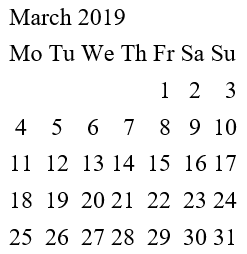Date and Time Function in Python || Functions in Python

# Date and Time Function in Python

0 2239

To keep the python language more light and compact, some section of libraries are divided into some modules that can be imported later as per requirement. Date and time functions are part of these modules. To work as a date objects for a date, Datetime module is used. These modules are used in various applications for tracking and playing with date and time in python language.

In the following Examples, we will learn how to import date, calendar, time module and all the basic concepts of date and time zone in the Python programming language.

Calendar Module
In python, the calendar module is used for calendar-related methods and functions in python. We can simply import the calendar module to display a calendar of the specified year. By default, it will take the first day of the week as Monday and whereas last day as a Sunday and if we want to do any modification in the calendar, this can be easily done with the help of one function i.e. calendar.setfirstweekday(). There is only need to call this function for updating any calendar module according to our requirements. There is a wide range of functions available in the calendar module with outstanding features.
How to see the calendar of the year in python

1. Firstly, we have to import the calendar.
import calendar

2. After that, a method of the calendar in which there needs to specify month and year of the calendar that we want to display in python. In the following example, I am writing code to display the calendar of the 3rd month of 2019.
calendarthird= calendar.month(2019,3)

3. The last step is to print that calendarthird
print(calendarthird)

Example

import calendar

calendarthird= calendar.month(2019,3)

print(calendarthird)

OutputHow to create date objects in python language
For creating any date object, the constructor of the datetime module and three parameters are required to be passed on the datetime().

Example
import datetime
dateobject = datetime.datetime(2017, 4, 20)
print(dateobject)

Output
2017-04-20 00:00:00

Time module
Displaying current time in python

Example
import time
timesystem=time.localtime(time.time())
print(timesystem)

Output
time.struct_time(tm_year=2018, tm_mon=11, tm_mday=23, tm_hour=7, tm_min=21, tm_sec=32, tm_wday=4, tm_yday=327, tm_isdst=0)

Display time in formatted form in python
import time;
localtime = time.asctime( time.localtime(time.time()) )
print "Local current time :", localtime

Output
Local current time : Fri Nov 23 07:29:55 2018
Datetime

Example
import datetime
d1=datetime.datetime.now()
print(d1)

Output
2018-11-23 07:34:42.018382

We can very easily formats data objects into the readable strings in python with the help of strftime() function that takes one parameter in it. Following are the list of some directives that are integrated with examples.

Example
import datetime
x = datetime.datetime(2020, 3, 2)
print(x.strftime("%a"))

Output
MonShare: